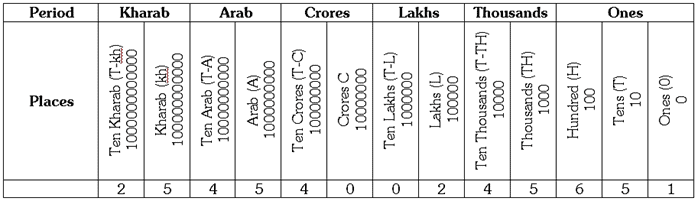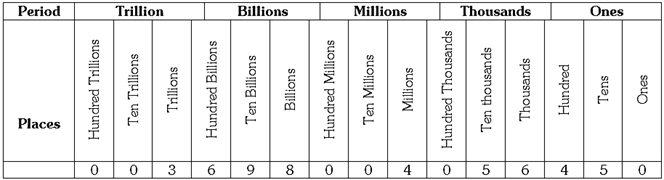Courses

# Test : Numbers - 2

## 10 Questions MCQ Test Mathematics for Class 5 (V) - CBSE and NCERT Curriculum | Test : Numbers - 2

Description
This mock test of Test : Numbers - 2 for Class 5 helps you for every Class 5 entrance exam. This contains 10 Multiple Choice Questions for Class 5 Test : Numbers - 2 (mcq) to study with solutions a complete question bank. The solved questions answers in this Test : Numbers - 2 quiz give you a good mix of easy questions and tough questions. Class 5 students definitely take this Test : Numbers - 2 exercise for a better result in the exam. You can find other Test : Numbers - 2 extra questions, long questions & short questions for Class 5 on EduRev as well by searching above.
QUESTION: 1

Solution:
QUESTION: 2

Solution:
QUESTION: 3

### In the Indian place value chart, which one of the following periods has been split into three places?

Solution:
QUESTION: 4

When a numeral is written as per the International system of numeration, the digit 5 is at the ten billions place in the numeral. What will be the place of the digit, if the numeral is written according to Indian system of numeration?

Solution:
QUESTION: 5

Name the number, indicated in the Indian place value chart, as per the International system of numeration.Solution:
QUESTION: 6

Which one of the following is the correct number for 7000000000 + 500000000 + 800000 + 600 + 9?

Solution:
QUESTION: 7

Name the number, indicated in the International place value chart, as per the Indian system of numeration.Solution:
QUESTION: 8

Which one of the following is not a member of natural numbers.

Solution:
QUESTION: 9

Write the number nineteen trillion seven hundred thirty five million eight hundred in expanded form.

Solution:
QUESTION: 10

X represents the five digit greatest number and Y represents 6 digit greatest number. Find the sum of X and Y.

Solution: